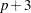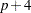The POWER Procedure
 ONECORR Statement

ONECORR <options> ;

The ONECORR statement performs power and sample size analyses for tests of simple and partial Pearson correlation between two variables. Both Fisher’s z test and thetest are supported.

Summary of Options

Table 68.6 summarizes categories of options available in the ONECORR statement.

Table 68.6 Summary of Options in the ONECORR Statement

Options

Define analysis

Specify analysis information

Specify effects

Specify sample size

Specify power

Control sample size rounding

Control ordering in output

Table 68.7 summarizes the valid result parameters in the ONECORR statement.

Table 68.7 Summary of Result Parameters in the ONECORR Statement

Analyses

Solve For

Syntax

TEST=PEARSON

Power

Sample size

Dictionary of Options

ALPHA=number-list

specifies the level of significance of the statistical test. The default is 0.05, corresponding to the usual 0.05100% = 5% level of significance. See the section Specifying Value Lists in Analysis Statements for information about specifying the number-list.

CORR=number-list

specifies the correlation between two variables, possibly adjusting for other variables as determined by the NPARTIALVARS= option. See the section Specifying Value Lists in Analysis Statements for information about specifying the number-list.

DIST=FISHERZ
DIST=T

specifies the underlying distribution assumed for the test statistic. FISHERZ corresponds to Fisher’snormalizing transformation of the correlation coefficient. T corresponds to thetransformation of the correlation coefficient. Note that DIST=T is equivalent to analyses in the MULTREG statement with NTESTPREDICTORS=1. The default value is FISHERZ.

MODEL=keyword-list

specifies the assumed distribution of the first variable when DIST=T. The second variable is assumed to have a normal distribution. MODEL=FIXED indicates a fixed distribution. MODEL=RANDOM (the default) indicates a joint bivariate normal distribution with the second variable. You can use the aliases CONDITIONAL for FIXED and UNCONDITIONAL for RANDOM. This option can be used only for DIST=T. See the section Specifying Value Lists in Analysis Statements for information about specifying the keyword-list.

FIXED

fixed variables

RANDOM

random (bivariate normal) variables

NFRACTIONAL
NFRAC

enables fractional input and output for sample sizes. See the section Sample Size Adjustment Options for information about the ramifications of the presence (and absence) of the NFRACTIONAL option.

NPARTIALVARS=number-list
NPVARS=number-list

specifies the number of variables adjusted for in the correlation between the two primary variables. The default value is 0, corresponding to a simple correlation. See the section Specifying Value Lists in Analysis Statements for information about specifying the number-list.

NTOTAL=number-list

specifies the sample size or requests a solution for the sample size with a missing value (NTOTAL=.). Values for the sample size must be at leastwhen DIST=T and MODEL=CONDITIONAL, and at leastwhen either DIST=FISHER or when DIST=T and MODEL=UNCONDITIONAL, whereis the value of the NPARTIALVARS option. See the section Specifying Value Lists in Analysis Statements for information about specifying the number-list.

NULLCORR=number-list
NULLC=number-list

specifies the null value of the correlation. The default value is 0. This option can be used only with the DIST=FISHERZ analysis. See the section Specifying Value Lists in Analysis Statements for information about specifying the number-list.

OUTPUTORDER=INTERNAL
OUTPUTORDER=REVERSE
OUTPUTORDER=SYNTAX

controls how the input and default analysis parameters are ordered in the output. OUTPUTORDER=INTERNAL (the default) arranges the parameters in the output according to the following order of their corresponding options:

The OUTPUTORDER=SYNTAX option arranges the parameters in the output in the same order in which their corresponding options are specified in the ONECORR statement. The OUTPUTORDER=REVERSE option arranges the parameters in the output in the reverse of the order in which their corresponding options are specified in the ONECORR statement.

POWER=number-list

specifies the desired power of the test or requests a solution for the power with a missing value (POWER=.). The power is expressed as a probability, a number between 0 and 1, rather than as a percentage. See the section Specifying Value Lists in Analysis Statements for information about specifying the number-list.

SIDES=keyword-list

specifies the number of sides (or tails) and the direction of the statistical test. Valid keywords are

1

one-sided with alternative hypothesis in same direction as effect

2

two-sided

U

upper one-sided with alternative greater than null value

L

lower one-sided with alternative less than null value

The default value is 2.

TEST=PEARSON

specifies a test of the Pearson correlation coefficient between two variables, possibly adjusting for other variables. This is the default test option.

Option Groups for Common Analyses

This section summarizes the syntax for the common analyses supported in the ONECORR statement.

Fisher’s z Test for Pearson Correlation

The following statements demonstrate a power computation for Fisher’s z test for correlation. Default values of TEST=PEARSON, ALPHA=0.05, SIDES=2, and NPARTIALVARS=0 are assumed.

```proc power;
onecorr dist=fisherz
nullcorr = 0.15
corr = 0.35
ntotal = 180
power = .;
run;
```

t Test for Pearson Correlation

The following statements demonstrate a sample size computation for the t test for correlation. Default values of TEST=PEARSON, MODEL=RANDOM, ALPHA=0.05, and SIDES=2 are assumed.

```proc power;
onecorr dist=t
npartialvars = 4
corr = 0.45
ntotal = .
power = 0.85;
run;
```Previous Page | Next Page | Top of Page